Geometry

# Surface Area: Level 2 Challenges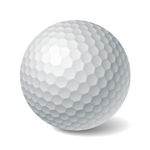In a spherical ball, a large number of dimples have been carved. The shape of these dimples is part of a sphere.

Is it possible for the dimpled ball to have a smaller surface area than it had before the dimples were carved?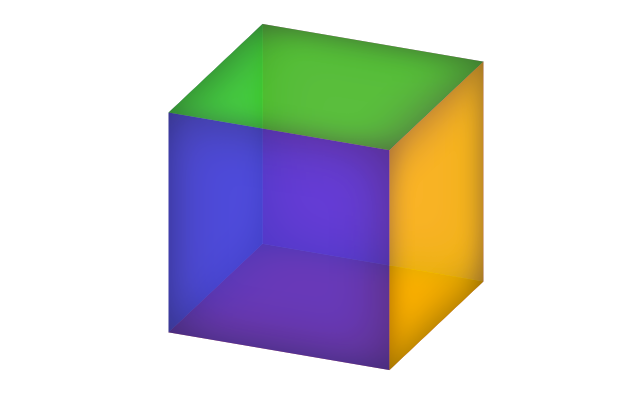The edge of a cube is increased by $20 \%$. What is the percentage increase in its surface area?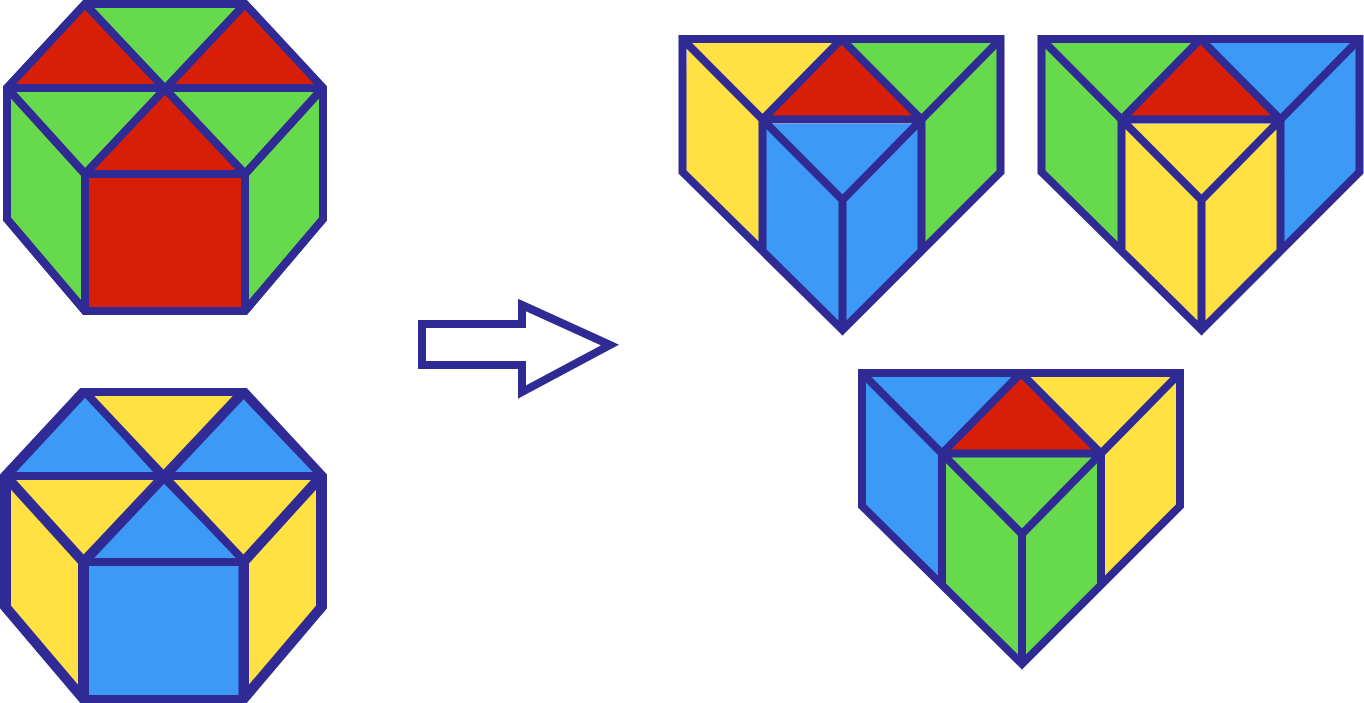Two equivalent regular hexagonal-based prisms are divided and rearranged into 3 equilateral triangular-based prisms, as shown above. After rearrangement, the total surface area of all prisms has increased by 12.5% with the constant height.

If the height of each prism is $\sqrt{3} \text{ cm}$, what is the side length of the regular hexagon base in $\text{cm}$?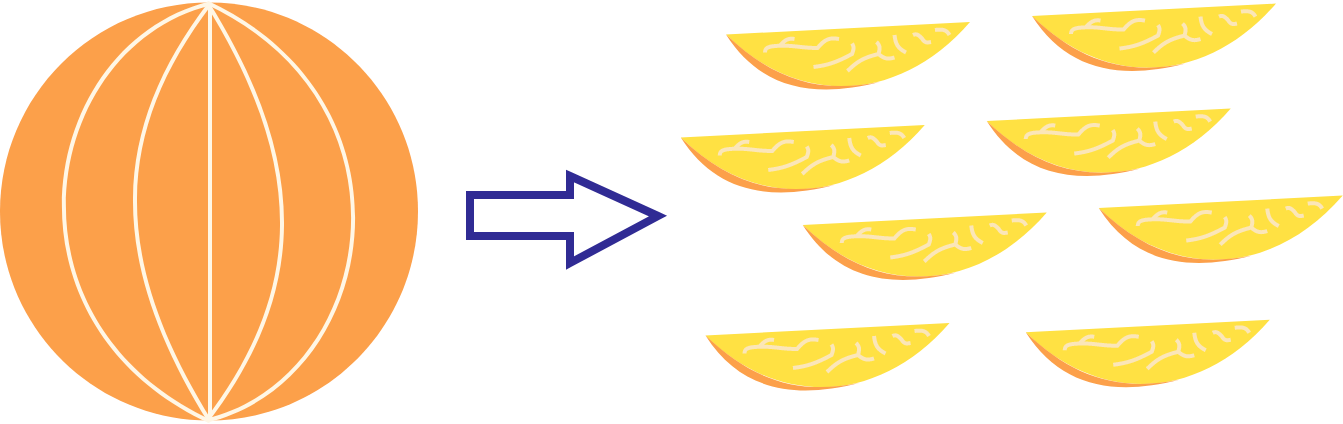A spherical orange is cut about the vertical axis into 8 equal slices as shown above.

What is the ratio of the total surface area of the 8 slices to that of the original orange?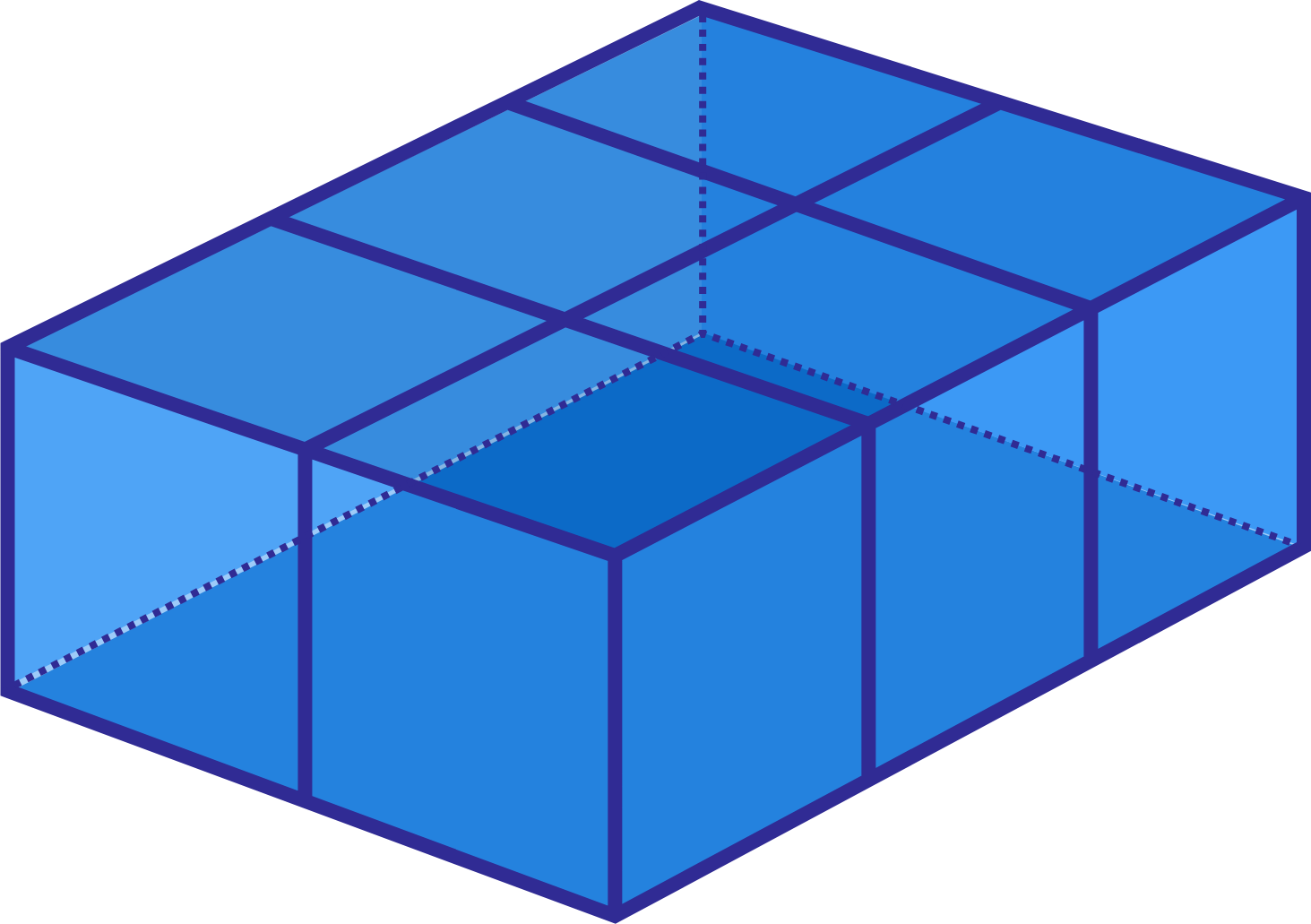If a cuboid of dimensions $10 \text{ cm} × 15 \text{ cm}× 5 \text{ cm}$ is cut to form cubes of sides 5 cm, then what is the difference between the sum of the surface areas of these cubes and the surface area of the original cuboid?

×